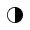• Resize textA -AA +
•# Seminar/ Colloquium

Home  »  Colloquium   »   Log-concavity of certain probability distributions.

## Log-concavity of certain probability distributions.Date: 27.10.2023 (Friday)
Time and Venue:  4.30-5.30 PM at the Ramanujan Hall (Transit Campus)
Speaker: Dr. Manjunath Krishnapur, Department of Mathematics, IISc Bangalore

Title: Log-concavity of certain probability distributions.
Abstract:  Log-concavity of a sequence (a_n^2\ge a_{n-1}a_{n+1}) or function (f(x)^2\ge f(x-h)f(x+h)) or measure (\mu(C)^2\ge \mu(A)\mu(B) if C=(A+B)/2)  gives many strong conclusions, such as its decay or `concentration’ properties. For example, the log-concavity of Lebesgue measure (known as Brunn-Minskowski inequality) implies the isoperimetric inequality.
In this lecture we show that many interesting distributions that arise in probability theory (particularly random matrix theory) are log-concave. We also give a partial solution to a problem of Chen that states that the distribution of the longest increasing sequence of a random permutation is log-concave. This is joint work with Jnaneshwar Baslingker and Mokshay Madiman. The talk is intended to be accessible to students with some knowledge of analysis and very basic notions of probability.
About the Speaker: Dr. Manjunath Krishnapur obtained PhD from the University of California, Berkeley in 2006. He has been with the Department of Mathematics, IISc Bangalore as a faculty member since 2009. His main area of research is probability theory.